# Using Prim’s Algorithm How To Find Minimum Spanning Tree?

In computer science, Prim’s algorithm is a greedy algorithm that finds a minimum spanning tree for a weighted undirected graph. This means it finds a subset of the edges that forms a tree that includes every vertex, where the total weight of all the edges in the tree is minimized. The algorithm was developed in 1930 by Czech mathematician Václav Matoušek and rediscovered in 1957 by computer scientist Robert C. Prim.

The algorithm is often used in networking and other applications where a minimum spanning tree is required. It is also a key part of Kruskal’s algorithm for finding the minimum spanning forest.

### MST:

A minimum spanning tree (MST) of an undirected graph is a spanning tree with a weight less than or equal to the weight of every other spanning tree. More generally, MST is used for a problem in which we are given a set of objects each with a weight, and we wish to connect the objects by some links so as to minimize the total weight of the resulting structure. The weight of a link is called its length.

We can use MST to model many problems in which we have to connect a set of objects with minimum total cost. For example, in an electrical network, the objects are the junctions (or nodes) and the links are the wires connecting them; the weights on the links represent the resistance of the wires. In this context, a spanning tree is a collection of wires that connect all the junctions with each other, without any loops (that is, it is acyclic). The MST problem is to find a spanning tree of minimum total resistance.

The MST problem can be solved using a variety of algorithms, including Kruskal’s algorithm, Prim’s algorithm, and Boruvka’s algorithm. In this article, we will focus on Prim’s algorithm.

## How does Prim’s Algorithm work?

Unlike Kruskal’s Algorithm, Prim’s algorithm starts with a random vertex and expands outward until it reaches all vertices in the graph. At each step, it adds the cheapest edge that connects the current tree to another vertex not yet in the tree.

The key to the algorithm is that it always expands the tree by adding the cheapest edge. This means that it will find the cheapest way to connect all the vertices together.

### Time Complexity:

The time complexity of Prim’s algorithm depends on the implementation, but it is typically O(ElogV), where E is the number of edges and V is the number of vertices.

There are several ways to implement Prim’s algorithm. One way is to use a binary heap to store the edges of the graph. The time complexity of this implementation is O(ElogV), where E is the number of edges and V is the number of vertices.

#### What is Binary Heap?

Binary heap is a data structure that allows you to efficiently find the minimum or maximum element from a set of elements.

A binary heap is a complete binary tree which satisfies the heap property. The heap property states that for any node N, the value of N is less than or equal to the values of its two children, if they exist.

Another way to implement Prim’s algorithm is to use a Fibonacci heap. The time complexity of this implementation is O(E + VlogV).

#### What is a Fibonacci Heap?

A Fibonacci heap is a heap data structure with a number of efficient operations. It is used in a number of algorithms such as Dijkstra’s algorithm, Prim’s algorithm, and Kruskal’s algorithm.

A Fibonacci heap is a heap composed of a collection of trees. Each tree is a min heap, meaning that the value of each node is less than or equal to the value of its children. In addition, each tree in the Fibonacci heap has a specific structure: it is either a single node, or it consists of two children, one of which is a min heap and the other is a max heap. This structure allows the Fibonacci heap to maintain a minimum value at the root of each tree.

Another way to implement Prim’s Algorithm is to use Priority Queue. Although using a priority queue does not gives us the optimal solution, but it is too easy to implement.

#### What is Priority Queue?

A priority queue is a type of queue in which each element has a “priority” associated with it. Priority queues are often implemented using heaps.

Elements in a priority queue are ordered according to their “priority”. Priority queues are typically implemented as min heaps, meaning that the element with the lowest priority is always at the root of the heap.

When an element is added to a priority queue, its priority is compared to the priorities of the other elements in the queue. The element is then inserted into the queue in such a way that the element with the highest priority is always at the root of the heap.

When an element is removed from a priority queue, the element with the highest priority is removed from the root of the heap.

Priority queues have a wide range of applications. They are commonly used in scheduling algorithms, such as those used in operating systems, and in graph algorithms, such as Dijkstra’s algorithm.

A priority queue can be implemented as either a max heap or a min heap. In a max heap, the element with the highest priority is always at the root of the heap. In a min heap, the element with the lowest priority is always at the root of the heap.

Now, let’s discuss the algorithm and the code. In this blog, we’re going to use Priority Queue, because it easier to implement than other other two.

### Pseudocode:

The pseudocode for the Prim’s algorithm is as follows:

Before starting the process, in Prim’s algorithm, we need to first do these two things.

1. Remove all the loops present in the graph.
2. Remove all the parallel edges.

Now start finding the MST.

2. Add v to the tree T.
3. Find the cheapest edge (v, w) that connects v to another vertex w, not in T.
4. Add the edge (v, w) to T.
5. Repeat steps 3-4 until all vertices are in T.

### Example:

Consider the following graph:

Our very first step is to check whether there is any loop or parallel edges. But in our given graph, there is no loop or parallel edges, so we’ll continue to our next step.

Taking any vertex as the root node:
We’re taking “A” as the root node.

Check the adjacent edges of the vertex “A”. Two edges are there. one is (A-B,4) and another is (A-D,8). We’ve to choose the minimum one, so we’ll choose (A-B,4).[Here, we’re writing the edge as (1st node – 2nd node, weight of the edge)]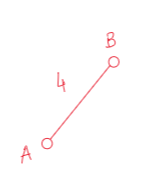Now node “B” is added the our MST. So now we’ll check all the adjacent edges of A and B. And we’ll take the edge with the minimum weight.
From node A there is only one edge we can find, i.e. (A-D,8).
From node B, there are total three edges.
1. (B-C,12)
2. (B-G,2)
3. (B-F,6)
So, we’ve to take the minimum weighted edge among all of them. 2 is the minimum, so we’ll take the edge, (B-G,2).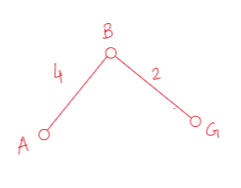Now, we’ve three nodes, A,B and G. So the total edges we’ve right now is 5.
1. (A-D,8)
2. (B-C,12)
3. (B-F,6)
4. (G-C,3)
5. (G-E,10)
Among them 3 is the minimum. So we’ll choose the edge (G-C,3).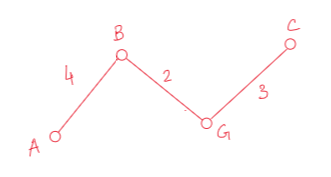Let’s see now how many edges we’ve.
1. (A-D,8)
2. (B-C,12)
3. (B-F,6)
4. (G-E,10)
5. (C-E,11)
6. (C-D, 7)
Among all these (B-F,6) edge is the minimum, so we’ll choose this one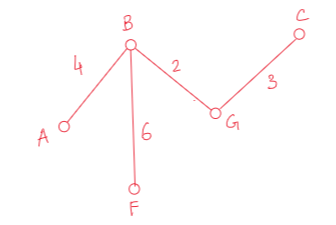We’re now very close to get our minimum spanning tree. Now we’ve total 6 edges to compare.
1. (A-D,8)
2. (B-C,12)
3. (G-E,10)
4. (C-E,11)
5. (C-D, 7)
6. (F-E, 5)
Obviously, (F-E, 5) is the minimum, so we’ll take this edge.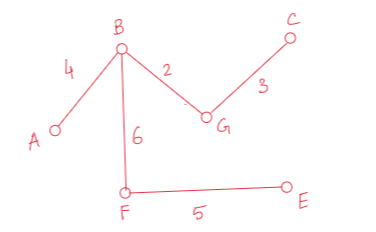Finally, we’ve only one node left to find the our minimum spanning tree.
1. (A-D,8)
2. (B-C,12)
3. (G-E,10)
4. (C-E,11)
5. (C-D, 7)
6.(E-D, 9)
Now, among these six edges, 5th one is the minimum, i.e. (C-D, 7). So we’ll take this edge.

Finally, we’ve found the minimum spanning tree of the following graph using Prim’s Algorithm. And the minimum cost of this spanning tree is =(4+2+3+6+5+7)=27.

The minimum spanning tree for this graph would be the following:

#### Code:

``````#include <stdio.h>

int a, b, u, v, n, i, j, ne = 1;

int visited = {0}, min, mincost = 0, cost;

int main(void)

{

printf("\nEnter the number of nodes:");

scanf("%d", &n);

for (i = 1; i <= n; i++)
{
for (j = 1; j <= n; j++)

{

scanf("%d", &cost[i][j]);

if (cost[i][j] == 0)

cost[i][j] = 999;
}
}

visited = 1;

printf("\n");

while (ne < n)

{

for (i = 1, min = 999; i <= n; i++)
{

for (j = 1; j <= n; j++)
{

if (cost[i][j] < min)

if (visited[i] != 0)

{

min = cost[i][j];

a = u = i;

b = v = j;
}
}
}

if (visited[u] == 0 || visited[v] == 0)

{

printf("\n Edge %d:(%d %d) cost:%d", ne++, a, b, min);

mincost += min;

visited[b] = 1;
}

cost[a][b] = cost[b][a] = 999;
}

printf("\n Minimum cost=%d", mincost);
}``````
##### Input:

For the input, we need to make the adjacent matrix first. Here is the adjacent matrix for the graph-

##### Output:

Edge 1:(1 2) cost:4
Edge 2:(2 7) cost:2
Edge 3:(7 3) cost:3
Edge 4:(2 6) cost:6
Edge 5:(6 5) cost:5
Edge 6:(3 4) cost:7
Minimum cost=27

##### Time Complexity:

This program has the time complexity O(N2).

## Application of Minimum Spanning Tree:

1. The MST problem can also be applied to a network of roads connecting a set of cities. In this context, the objects are the cities, the links are the roads connecting them, and the weights on the links represent the lengths of the roads. The MST problem is to find a set of roads of minimum total length that connect all the cities.
2. The MST problem can also be applied to a network of computers connected by communication links. In this context, the objects are the computers, the links are the communication links connecting them, and the weights on the links represent the bandwidths of the communication links. The MST problem is to find a set of communication links of minimum total bandwidth that connect all the computers.
3. The MST problem can also be applied to a network of pipes connecting a set of houses. In this context, the objects are the houses, the links are the pipes connecting them, and the weights on the links represent the diameters of the pipes. The MST problem is to find a set of pipes of minimum total diameter that connect all the houses.

There are many other applications of MST. In general, MST can be applied to any problem in which we have a set of objects and we wish to connect them so as to minimize the total cost of the resulting structure.

So that’s all for this blog.

See you in the next blog.